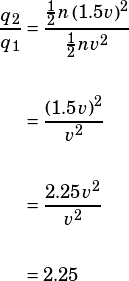Can you do test 4 section 4 number 35 please?

This is a very SAT-ish question: the math is pretty trivial, but conceptually the question is still a bit tricky.

There are a couple things you need to have nailed down to get this one right.

First, you need to recognize that they’re asking you for a ratio of two dynamic pressures, so they’re asking you for a ratio of two q‘s. Let’s call themand. We’ll sayis the one that corresponds to a velocity of v andis the one that corresponds to a velocity of 1.5v.

Second, you need to make sure you’re providing the ratio that’s asked for: the q of the faster fluid to the q of the slower fluid. Which fluid is faster—the one with velocity v or the one with velocity 1.5v? 1.5v is always going to be a larger number than v, so that’s the faster fluid. Therefore, we need to calculate the ratio ofto.